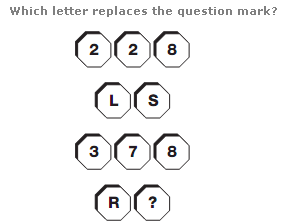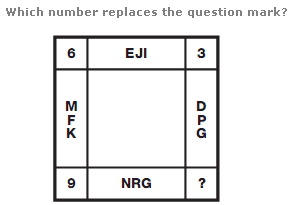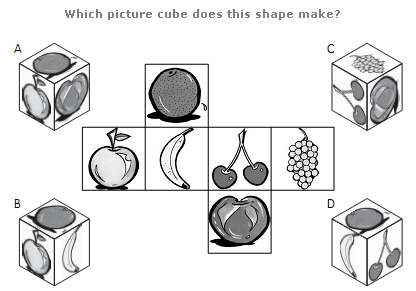# Puzzles - Logical puzzles

### Exercise :: Logical puzzlesAnswer : U Explanation : Multiply the numerical values of the letters in each pair to give the 3 digit result in the spaces above.

View Answer & ExplanationAnswer : 12 Explanation : The value at each corner of the diagram equals the difference between the sums of the numerical values of the letters in the boxes adjacent to the corner.

View Answer & ExplanationAnswer : C Explanation : [NIL]

View Answer & Explanation

#### Current Affairs 2019

Interview Questions and Answers